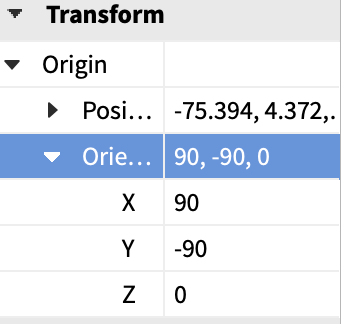# How to get orientation of model

how can I get the orientation under transform in the properties from a script?2 Likes
``````game.Workspace.Model.Part.Rotation
``````

Models don’t have an Orientation property because they are just a group of Parts. If you need a Model’s rotation, get the rotation of one of the parts inside the model.

1 Like

Models have a function called :GetBoundingBox() which returns the models BoundingBox CFrame and Size

``````local cframe,size = model:GetBoundingBox()
``````
2 Likes
``````local cframe = model:GetPivot()
local rx, ry, rz = cframe:ToOrientation()
``````
3 Likes

@Dan_E56 @bronsonman
The solution you provide me is getting the model position not the orientation. I need the orientation to rotate the model in the Y axis.

1 Like

You can get the orientation from the cframe it gives you by doing cframe:ToOrientation()

1 Like

To get this specific Orientation you do PVInstance:GetPivot():ToOrientation()

2 Likes

Error i Get is PVInstance is not a valid member of Model “Workspace.Modelz”

Replace PVInstance with your model, sorry for the confusion.

1 Like

It keeps printing numbers that are not the orientation example
my models orientation numbers 34.393, 96.933, 12.148
it prints 0.6002667546272278

1 Like

it is still printing the position not orientation

1 Like

This is unlikely, it is likely returning the orientation in the format other than degrees, if you want to convert these values to degrees you can use math.deg.

I have tried this Devfourm already issue was I couldn’t rotate the model using the orientation ( it does print it out correctly though)

1 Like

Could you show me where you are rotating the model?

Sure!
Code

``````game.Players.PlayerAdded:Connect(function(plr)
local m = game.Workspace.Modelz
local X, Y, Z = m:GetPivot():ToOrientation()
local OrientationY	= math.deg(Y)
print(OrientationY)
OrientationY += 24
print(OrientationY)
``````

When I add the 24 to the Y axis it doesn’t change in the properties or rotate it but it will print the + 24 to the y axis orientation.

This doesn’t show where you are setting the rotation of the model

What do you mean by setting the rotation of model? Here is a screen shot of the rotation hope this is what you mean?

What I meant was the line of code?

Ohh, the code I sent was the whole script (I am now second guessing myself that I wrote it incorrectly)

``````game.Players.PlayerAdded:Connect(function(plr)
local m = game.Workspace.Modelz
local X, Y, Z = m:GetPivot():ToOrientation()
local OrientationY	= math.deg(Y)
print(OrientationY)
OrientationY += 24 -- wouldn't this add 24 to the y axis so it would move by 24
print(OrientationY)

end)
``````
1 Like

Are you expecting this to change the Y orientation of the model? To update the orientation of the model you need to use :PivotTo()

2 Likes